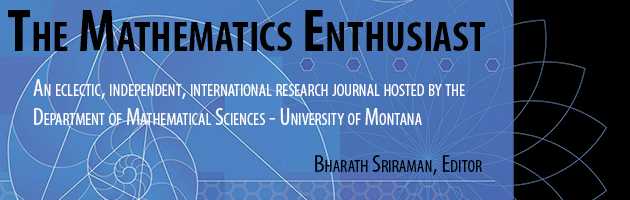•
•19

1

#### Abstract

In this theoretical essay we problematize the statement “To teach mathematics one must know a specific mathematics for teaching” of the discourse of specific mathematical knowledge to teach. The study was inspired by Foucaultian concepts and the analysis focused on studies in the area of Mathematical Education that mobilize discourses regarding Mathematical Knowledge for Teaching, Mathematics Teacher’s Specialized Knowledge and Mathematics for Teaching. The analysis showed that the discourses operate under two truth regimes about the mathematical knowledge needed to teach. Analyzing the enunciative intertwining, we observe an approximation with: i) the educational discourse, which deals with the inseparability between theory and practice; and ii) the capitalist economic discourse, which associates of economic development with the mathematical proficiency of a country.

136

157

COinS